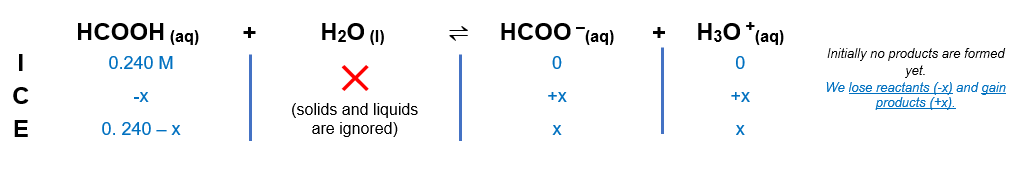# Problem: Determine the [H3O+] of a 0.240M solution of formic acid.

###### FREE Expert Solution

Formic acid is weak so we have to use an ICE chart to solve.

We’re being asked to calculate the pH of a 0.240 M aqueous solution of HCOOH.

HCOOH(aq) + H2O(l)  H3O+(aq) + HCOO(aq); Ka = 1.8 × 10–4

From this, we can construct an ICE table. Remember that liquids are ignored in the ICE table.94% (179 ratings)###### Problem Details

Determine the [H3O+] of a 0.240M solution of formic acid.